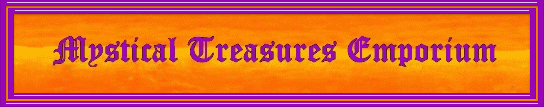CURRENT MOONConvert Words and Names to Numbers

One method of converting letters and words to a numeric value is to use a simple translation table that assigns each letter to the numbers 1 through 9 as shown below. As you will note, case does not matter.

 1 2 3 4 5 6 7 8 9 A, a B, b C, c D, d E, e F, f G, g H, h I, i J, j K, k L, l M, m N, n O, o P, p Q, q R, r S, s T, t U, u V, v W, w X, x Y, y Z, z

To use this method performt the following steps:
1. Translate all letters to numbers
2. Add the value of all the numbers
3. If the resulting value is more than 1 digit then add the digits together and repeat until only one digit remains

The following calculator will perform the calculations for you. Just enter the desired word(s) or name and click on the Calculate button. Press the back button of your browser to perform another calculation.